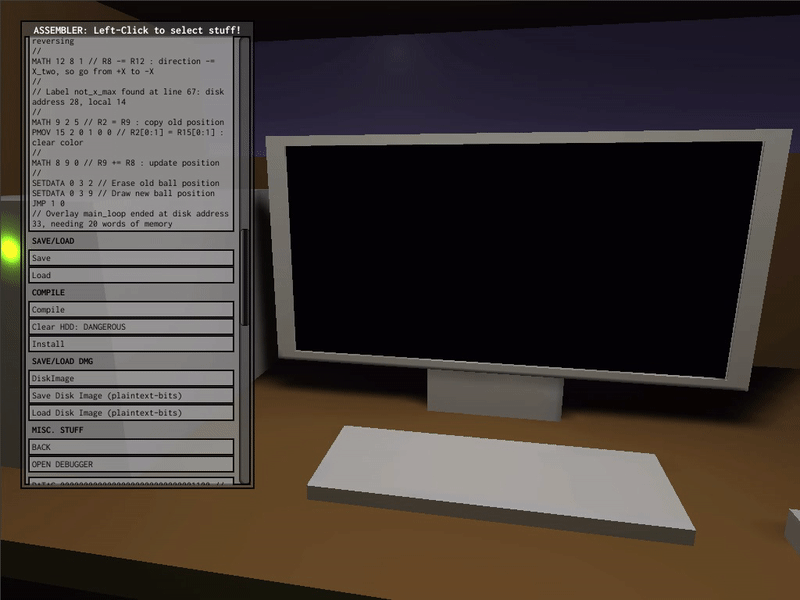Viewing post in Code sharing megathread

(2 edits) (+1)

Here's a classic screensaver - the bouncy ball. While written as a couple overlays using my overlay loader, it's small enough that it would fit in ROM with only a little rearranging of the code. I haven't managed to get it quite small enough to be loaded from disk as one unit by the built-in bootloader though, even by making assumptions on the start-up values of registers it takes a word or two too many. Might be possible with some tricks, but I haven't quite gotten there. (Edit: Not sure why I bothered, but I figured it out, so here's the code for a version that the built-in bootloader can (barely) load.)Here's the code:

OVERLAY init
MOVI 13 @local:y_two
MOVI 12 @local:x_two
MOVI 9 @local:pos
MOVI 8 @local:dir
MATH 3 3 1
MATH 2 2 1
SET 2 3 @overlay:main_loop
JMP 3 9
pos: DATAC 0b11_1000_100...
dir: DATAC 0b00_0001_001...
y_two: DATAC 0b00_0000_010...
x_two: DATAC 0b00_0010_000...
OVERLAY main_loop
loop:
MATH 2 2 1
PMOV 9 2 6 8 23 1 // R2 = Y pos
SET 3 3 7 // R3 = Y max
IFJMP 1 @local:not_y_max 1 // if not at Y_max, skip reversing
MATH 13 8 1 // R8 -= R13 : direction -= Y_two, so go from +Y to -Y
not_y_max:
SET 3 3 0 // R3 = Y min
IFJMP 1 @local:not_y_min 1 // if not at Y_min, skip reversing
MATH 13 8 0 // R8 += R13 : direction += Y_two, so go from -Y to +Y
not_y_min:
PMOV 9 2 2 5 26 1 // R2 = X pos
// SET 3 3 0 : R3 = X min : R3 is already 0
IFJMP 1 @local:not_x_min 1 // if not at X_min, skip reversing
MATH 12 8 0 // R8 += R12 : direction += X_two, so go from -X to +X
not_x_min:
SET 3 3 15 // R3 = X max
IFJMP 1 @local:not_x_max 1 // if not at X_max, skip reversing
MATH 12 8 1 // R8 -= R12 : direction -= X_two, so go from +X to -X
not_x_max:
MATH 9 2 5        // R2 = R9 : copy old position
PMOV 15 2 0 1 0 0 // R2[0:1] = R15[0:1]  : clear color
MATH 8 9 0        // R9 += R8 : update position
SETDATA 0 3 2     // Erase old ball position
SETDATA 0 3 9     // Draw new ball position
JMP 1 @local:loop

EDIT: Gah. The code has empty lines to make it clearer which lines go together, but this site's post editor apparently doesn't like that... (Yet another annoyance to add to the list about it I guess...)